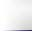Home View Cart Tech Info Projects ContactTech Info: Physics Theory#### Electrical Power

The flow of electricity can be thought of as similar to the flow of liquid in a hose, where voltage is the amount of pressure and current is the rate of flow. Bigger hoses have less resistance, so more liquid will flow for a given pressure. Similarly in electronics, lower resistances result in higher current flow at a given voltage. The relationship between voltage, current and resistance is known as Ohm's law, as follows:

 Voltage = Current x Resistance      or V = IR

The amount of electrical power flowing through a circuit is simply the product of the voltage times the current:

 Power = Voltage x Current    or P = VI

Substituting in ohm's law into the second equation, we can see that the power lost by a circuit is equal to its resistance times the square of the current:

 P = I²R

This is important because in the case of a motor, doubling voltage results in twice the current flow, so power is increased by a factor of 4. But power loss (usually in the form of heat) also increases by a factor of 4 as well.

#### Energy Storage

Batteries typically express their capacity in Amp-Hours (Ah). For example, if a battery is rated to 100Ah, it means you can draw 100 amps from it for 1 hour before it goes flat. Double the current, and it will take half as long to go flat, etc.

Sometimes you will come across C ratings for batteries, particularly referring to discharge rates. C simply represents the capacity of the battery in Ah, so if a 100Ah battery is rated to 5C, it means you can draw a maximum of 500 amps from it.

Sometimes it is more useful to consider a battery's capacity in watt-hours, since it is more representative of the actual stored energy. To calculate this, simply multiply the amp-hour rating by its nominal voltage. To convert watt hours to joules, multiply by 3600 (the number of seconds in an hour, since 1 watt = 1 joule per second).

#### Aerodynamic Drag and Rolling Resistance

The amount of power a vehicle needs in order to travel at a given speed can be approximately calculated by adding the aerodynamic drag and rolling resistance. Aerodynamic drag may be calculated using the following formula:

 Pdrag = 0.5 ρ Cd A V³ Pdrag = aerodynamic drag ρ = density of air, 1.2 kg/m³ Cd = coefficient of drag A = frontal area of vehicle, in m² V = velocity of vehicle, in m/s

And rolling resistance may be calculated as follows:

 Prr = V Crr g m Prr = rolling resistance V = velocity, in m/s Crr = coefficient of rolling resistance g = gravitational constant, 9.81 m/s⊃ m = mass of vehicle, in kg

For example, at 60km/h (16.7m/s) a compact 1000kg vehicle with good tires will have

• Aerodynamic drag of 0.5 x 1.2 x 0.3 x 2 x 16.7³ = 1676W.
• Rolling resistance of 16.7 x 0.01 x 9.81 x 1000 = 1638W

So, total power required to maintain the vehicle's speed at 60 km/h is about 3.3kW, or 4.4 hp. The other factor to consider is transmission losses, which is typically between 10% (direct drive setups) to 30% (such as all wheel drive vehicles).

#### Vehicle Efficiency

The efficiency of an electric vehicle is usually measured in watt-hours per km (or mile), i.e the average amount of energy required to drive the car a given distance.

A typical EV might have a 15kWh pack (1C rating), and have a 100 km range. Therefore it has an efficiency of 15000/100 or 150wh/km.

Highly efficient vehicles may go as low as 100wh/km. Larger vehicles such as SUVs may be as high as 400wh/km. Aerodynamic drag and rolling resistance (see above) are the primary factors influencing this.

#### Wire Gauge Information

Most electrical wire/cable used for the power lines in EVs is rated by the American Wire Gauge, or AWG. The most common size used is 2/0 from battery to speed controller and 4/0 from speed controller to motor, though of course requirements will vary depending on the system's specifications. The table below outlines the specifications of the larger cable gauges. Note that current ratings are continuous, cables can usually withstand much higher currents for short periods.

AWG

Diameter
(mm)

Turns of wire
per cm
Area
(mm²)
Copper Resistance
(ohms per km)
Copper wire
current rating
with 60 C insulation
000000(6/0)
14.73
0.68
170
0.10
400A
00000(5/0)
13.12
0.76
135
0.13
300A
0000(4/0)
11.68
0.85
107
0.16
250A
000(3/0)
10.4
0.96
85
0.2
200A
00(2/0)
9.266
1.08
67.4
0.25
160A
0(1/0)
8.251
1.21
53.5
0.33
130A
1
7.348
1.36
42.4
0.4
110A
2
6.544
1.53
33.6
0.5
95A
3
5.827
1.72
26.7
0.65
85A
4
5.189
1.93
21.2
0.8
70A#### Units

 Metric Symbol Unit Voltage V Volts, V Current I Amperes, A Resistance R Ohms, Ω Power P Watts, W Energy E Joules, J Capacitance C Farads, F Inductance L Henry, H

#### Unit Converter

 Convert: Please select.. Distance Energy Power Speed Torque Weight

Zero Emission Vehicles Australia © 2020 :: Terms and Conditions, Privacy Policy, Payments and Delivery, Warranty and Returns